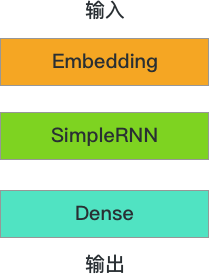## 深度学习：循环神经网络构建

3682 字 · 154 阅读 · 2023 年 06 月 04 日• IMDB 数据集
• 词嵌入
• 简单循环神经网络
• LSTM 循环神经网络

### IMDB 数据集# 从镜像服务器下载数据集
keras_dir = "/root/.keras/datasets"
wget -nc -P $keras_dir "http://labfile.oss.aliyuncs.com/courses/1081/imdb.npz" wget -nc -P$keras_dir "http://labfile.oss.aliyuncs.com/courses/1081/imdb_word_index.json"

import numpy as np
import tensorflow as tf

# 加载数据, num_words 表示只考虑最常用的 n 个词语，代表本次所用词汇表大小
MAX_DICT = 1000
(X_train, y_train), (X_test, y_test) = tf.keras.datasets.imdb.load_data(
num_words=MAX_DICT)

X_train.shape, y_train.shape, X_test.shape, y_test.shape


np.array(X_train)  # 直接运行


index = tf.keras.datasets.imdb.get_word_index()  # 获取词索引表
reverse_index = dict([(value, key) for (key, value) in index.items()])
comment = " ".join([reverse_index.get(i - 3, "#")
for i in X_train])  # 还原第 1 条评论
comment


MAX_LEN = 200  # 设定句子最大长度

X_train.shape, X_test.shape


### 词嵌入tf.keras.layers.Embedding(input_dim, output_dim, embeddings_initializer='uniform', embeddings_regularizer=None, activity_regularizer=None, embeddings_constraint=None, mask_zero=False, input_length=None)



- input_dim：int > 0，词汇表大小。

- output_dim：int >= 0，词向量的维度。

- input_length：输入序列的长度，当它是固定的时候。如果你需要连接 Flatten 和 Dense 层，则这个参数是必须的。



model = tf.keras.Sequential()

model.summary()


model.compile(optimizer='Adam', loss='binary_crossentropy',
metrics=['accuracy'])


EPOCHS = 1
BATCH_SIZE = 64
model.fit(X_train, y_train, BATCH_SIZE, EPOCHS,
validation_data=(X_test, y_test))


### 简单循环神经网络

tf.keras.layers.SimpleRNN(units, activation='tanh', use_bias=True, kernel_initializer='glorot_uniform', recurrent_initializer='orthogonal', bias_initializer='zeros', kernel_regularizer=None, recurrent_regularizer=None, bias_regularizer=None, activity_regularizer=None, kernel_constraint=None, recurrent_constraint=None, bias_constraint=None, dropout=0.0, recurrent_dropout=0.0, return_sequences=False, return_state=False, go_backwards=False, stateful=False, unroll=False)



- units: 正整数，输出空间的维度。

- activation: 要使用的激活函数。如果传入 None，则使用线性激活。

- use_bias: 布尔值，该层是否使用偏置项量。

- dropout: 在 0 和 1 之间的浮点数。

- return_sequences: 布尔值。是返回输出序列中的最后一个输出，还是全部序列。model_RNN = tf.keras.Sequential()
# dropout 是层与层之前的 dropout 数值，recurrent_dropout 是上个时序与这个时序的 dropout 值
dropout=0.2,
recurrent_dropout=0.2))

model_RNN.summary()


model_RNN.suammry() 可以帮我们清晰地看出模型结构，模型总共要学的参数数量较大。接下来，我们对模型进行编译和训练，并最终输出模型在测试集上的评估情况。

model_RNN.compile(optimizer='Adam', loss='binary_crossentropy',
metrics=['accuracy'])
model_RNN.fit(X_train, y_train, BATCH_SIZE, EPOCHS,
validation_data=(X_test, y_test))


### LSTM 循环神经网络

model_LSTM = tf.keras.Sequential()
dropout=0.2,
recurrent_dropout=0.2))

model_LSTM.summary()


model_LSTM.summary() 的输出中我们看到，LSTM 比起简单 RNN 会多学到一些参数，但这些参数帮助我们规避了梯度消失等致命性问题。我们保持其他几层神经网络不变，LSTM 的两个 dropout 与 SimpleRNN 的两个 dropout 值一致。

model_LSTM.compile(optimizer='Adam', loss='binary_crossentropy',
metrics=['accuracy'])
model_LSTM.fit(X_train, y_train, BATCH_SIZE, EPOCHS,
validation_data=(X_test, y_test))


model_GRU = tf.keras.Sequential()
dropout=0.2,
recurrent_dropout=0.2))

model_GRU.summary()

model_GRU.compile(optimizer='Adam', loss='binary_crossentropy',
metrics=['accuracy'])
model_GRU.fit(X_train, y_train, BATCH_SIZE, EPOCHS,
validation_data=(X_test, y_test))


### 系列文章•